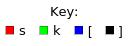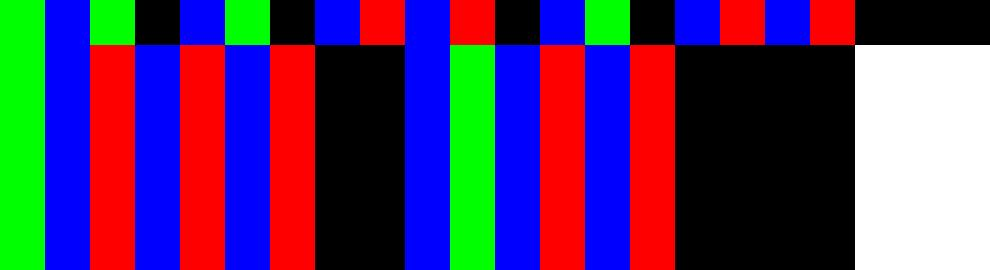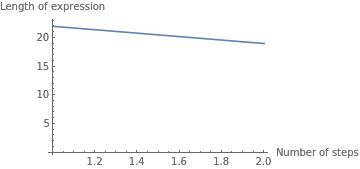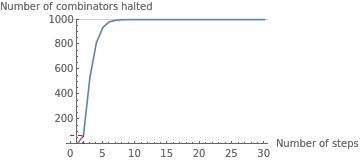## SK Combinator Expression Analysis

Input a valid SK combinator expression or use the random expression above.

Expression:
k[k][k][s[s][k][s[s]]]

Visualisation:
 Initial state and first 5 steps of evaluation: The nth row of the image represents the nth step of evaluation of the combinator.Graph of Evaluated Expression Lengths:
 As the combinator is evaluated, its length changes. This graph plots length of combinator against number of steps of evaluation.Statistical Analysis of Halting Time:
 This combinator has 8 leaves. This combinator falls within the 7th percentile of a random sample of 1000 combinators with leaf size 8, halting after 2 steps - on average, 7.1% of combinators from this sample halt before this one. A graph demonstrating this follows:Machine Learning Analysis of Halting:
 A machine learning model (using logistic regression) has been trained on a sample of 1456 rasterised images of the first 5 steps of random SK combinator expressions with varying leaf lengths, achieving 87.7% accuracy when classifying a test set of 1454 random SK combinator expressions as halting or non halting (before 40 steps). This particular expression, which halts after 2 step(s), is classified as having a Indeterminate% probability of halting, and a Indeterminate% probability of not halting (before 40 steps).

Evaluated expression:
Halted in 1 step(s)
 0 k[k][k][s[s][k][s[s]]] 1 k[s[s[s]][k[s[s]]]]